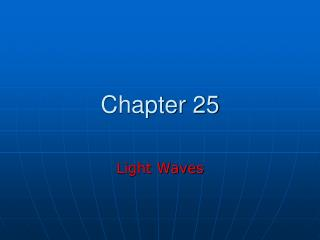Download PresentationChapter 25Chapter 25 - PowerPoint PPT Presentation

Download PresentationChapter 25
An Image/Link below is provided (as is) to download presentation

Download Policy: Content on the Website is provided to you AS IS for your information and personal use and may not be sold / licensed / shared on other websites without getting consent from its author. While downloading, if for some reason you are not able to download a presentation, the publisher may have deleted the file from their server.

- - - - - - - - - - - - - - - - - - - - - - - - - - - E N D - - - - - - - - - - - - - - - - - - - - - - - - - - -
Presentation Transcript

1. Chapter 25 Light Waves

2. C = f l C: speed of light C = 3 x 108 m/s in vacuum EM wave can travel in the absence of medium. In other medium, the speed of light is smaller than in vacuum.

3. 3 x 108 = f l nano = 10-9 http://laxmi.nuc.ucla.edu:8248/M248_99/iphysics/spectrum.gif

4. Human eye is sensitive 380 < l < 700 nm l < 300 nm (UV) is absorbed mostly by cornea and l > 1200 nm by aqueous humor. Green cell (most sensitive for 520 nm), and 2 other types of cells sensitive to shorter and longer wavelength sense light and send signal to brain.

5. Light Intensity All electromagnetic waves such as light transmit energy. Light intensity = power flowing through the area / area (power density) [w/m2] Q. A laser sends out a beam 3 mW power. The diameter of the beam is 3 mm. What is the intensity of the laser beam in w/m2? Intensity = (0.003 w)/(p x 0.00152 m2) = 424.4 w/m2 My laser point produces about 5 mW power!!!

6. When a light bulb is on, it radiates out broad spectrum of EM wave. The total radiant energy emitted by the lamp per unit time is called radiant flux. Only a small portion of this is in visible range (400 – 700 nm). The portion of radiant energy in visible range is called luminous flux (F). In a incandescent light bulb, only 10% is luminous flux. 1 lumen (lm) = light power of 1/683 W in yellow (lighting industry)

7. Solid Angle 2 Dimension s • = s/R [radian]  R Total solid angle: q = 2pR/R = 2p (rad) A W = A/R2 [steradian] W R Total solid angle: W = 4pR2/R2 = 4p (sr)

8. W R1 R2 Q. What solid angle is subtended at the center of 6 m diameter sphere by a 2 m2 area on its surface? W = A/R2 = (2 m2)/(3 m)2 = 0.22 sr Luminous intensity: I = F/W (luminous flux per unit solid angle) [lm/sr] = candela (cd)

9. 3 m2 10 m Q. A spotlight is equipped with a 30 cd bulb. This light beams onto a vertical wall in a circular shape with 3 m2 area. The spotlight is 10 m from the wall. Calculate the luminous intensity for the spotlight. Total luminous flux coming out of the bulb, F = I 4p = (30 cd)(4p sr) = 377 lm This amount of luminous flux is concentrated on the 3 m2 spot. To get the luminous intensity, we need to know the solid angle that bright spot subtend. W = A/R2 = (3 m2)/(10 m)2 = 0.03 sr I (spot) = (377 lm)/(.03 sr) = 1.26 x 104 cd

10. 2 1 W R1 R2 Illuminance E = F/A Luminous flux per unit area [lm/m2] = Lux (lx) I1 = I2 However, E1 ≠ E2 E1 = F/A1 = F/(WR12), E2 = F/A2 = F/(WR22) W = A/R2

11. A q W = Acos/R2 I = F/W = F/(Acosq/R2) E = F/A E = Icos/R2 Relation between I (luminous intensity) and E (illuminance) I = F/W E = F/A W = A/R2 E = I/R2

12. q q Q. A 300 cd lamp is located 3 m from a surface with 0.25 m2 area. The luminous flux makes an angle of 30 deg with the normal to the surface. (a) What is the illuminance? (b) what is the luminous flux shining on the surface? • Flux thru the surface: f • f = I W W = A/R2 • = (0.25)cos30/32 • = 0.024 sr f = (300 cd)(0.024 sr) = 7.2 lm E = f/A = (7.2 lm)/(0.25 m2) = 28.8 lx

13. http://atom.physics.calpoly.edu/Graphics/em_surface.gif

14. x z y E B Polarization + - For this type of EM wave, E field oscillates in a fixed direction, and so does B field. Linearly polarized!! Direction of E-field determines polarization direction: This wave is polarized in x-direction.

15. E Natural light is “unpolarized”. We can produce polarized light from unpolarized light!! Polaroid sheet allows light pass through inly if the E-field vector is in a specific direction.

16. For unpolarized light, a polaroid sheet reduces its intensity to half (sunglasses).